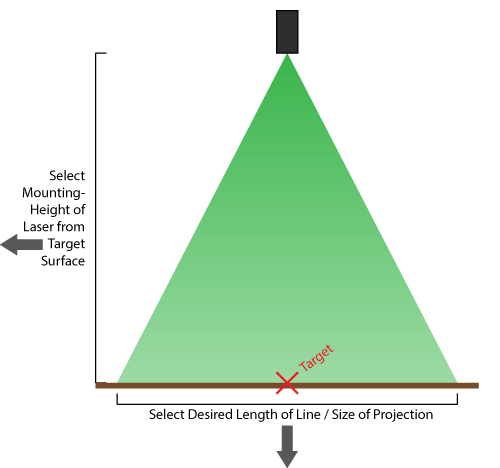## Fan Angle Calculator

 To achieve your desired projection, select a minimum fan angle of: 67 degrees Meters Centimeters Feet Inches 37m49m Meters         Centimeters         Feet         Inches

## Divergence Calculation

 Distance [m]: Laser: Power [mW]: Divergence [mrad]: Diameter at aperture [mm]:
 Beam diameter after m: mm Area of laser point after m: mm2 Laser intensity of uniform beam after m: mW/mm2

## Find a laser beam's divergence

 Specifications: Distance from Laser Aperture -> Projection surface [m]: Beam Waist Diameter at Laser Aperture [mm]: Laser spot diameter at the projection surface [mm]:
 The Full Divergence of the laser system is: 0.00 mrad

## Calculating nanometers from wavenumber

To convert from wavenumber (cm-1) to nanometers, use the following equation: 1/cm-1 x 107 = nanometers. Or to convert from nanometers to wavenumber, you simply reverse the equation so that wavenumber (cm-1) = 107 / wavelength in nanometers. Or use the tool below.

## Converting angstroms to nanometers

To convert from angstroms to nanometers, use the following equation:

1 angstrom = 0.1 nanometer. Or use the tool below.

## Converting fahrenheit to celsius

To convert from fahrenheit to celsius, use the following equation:

32° Fahrenheit = 0° Celsius. Or use the tool below.

## Converting watts to joules

Power is measured in Watts, named after James Watt (1736-1819). Joules (named after James Prescott Joule, 1818-1889) are units of energy. Use the following equation to convert from Watts to Joules.

1 Watt = 1 Joule per second of power

## Calculating duty factor

Duty factor (also known as duty cycle) is the ratio of pulse duration to pulse period. Duty factor (Df) is calculated as follows.

Df = pulse duration (sec) / pulse repetition period (sec)

Multiply the result by 100 to get your answer as a percentage.

Please note that as pulse repetition frequency increases, duty factor increases. As pulse repetition period increases, duty factor decreases. As pulse duration increases, duty factor increases.

## Converting wavelength to frequency

Use the following equation to convert wavelength to frequency: v (Hz) = 2.998 x 1017 / wavelength (nm).

## Converting wavenumber to frequency

Use the following equation to convert wavenumber to frequency: v (Hz) = 2.998 x 1010 x wavenumber (cm-1)